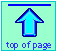Note: this document will print in an appropriately modified format (14 pages)

1  Activity series of the metals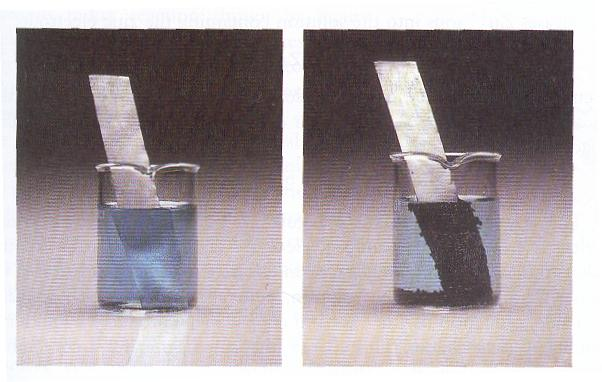It has long been known that some metals are more "active" than others in the sense that a more active metal can "displace" a less active one from a solution of its salt. The classic example is the one we have already mentioned on the preceding page:

Zn(s) + Cu2+ → Zn2+ + Cu(s)

Here zinc is more active because it can displace (precipitate) copper from solution. If you immerse a piece of metallic zinc in a solution of copper sulfate, the surface of the zinc quickly becomes covered with a black coating of finely-divided copper, and the blue color of the hydrated copper(II) ion diminishes.

Similar comparisons of other metals made it possible to arrange them in the order of their increasing electron-donating (reducing) power. This sequence became known as the electromotive or activity series of the metals.

This table shows only some of the more common elements of the activity series.

The most active (most strongly reducing) metals appear on top, and least active metals appear on the bottom.

A more active metal (such as Zn) will donate electrons to the cation of a less active metal (Cu2+, for example.)

Notice the special role of hydrogen here; although H2 does not have the physical properties of a metal, it is capable of being "displaced" from H2O or H+-containing (acidic) solutions. Note that al all the metals above hydrogen are capable of donating electrons to H+.

Metals that appear below hydrogen are not strong enough electron donors to reduce hydrogen ions. These "oxididation" reactions run in the reverse direction (notice the left-pointing arrows), so it is now the H2 that is oxidized.

Activity series of the metals
Observed action of metal   reaction equation
displaces H2 from water, steam, or acids Li 2 Li(s) + 2 H2O(l) → 2 LiOH(aq) + H2(g)
K 2 K(s) + 2 H2O(l) → 2 KOH(aq) + H2(g)
Ca Ca(s) + 2 H2O(l) → Ca(OH)2(s) + H2(g)
Na 2 Na(s) + 2 H2O(l)) → 2 NaOH(aq) + H2(g)
displaces H2 from steam or acids Mg Mg(s) + 2 H2O(g) → Mg(OH)2(s) + H2(g)
Al 2 Al(s) + 6 H2O(g) → 2 Al(OH)3(s) + 3 H2(g)
Mn Mn(s) + 2 H2O(g) → Mn(OH)2(s) + H2(g)
Zn Zn(s) + 2 H2O(g) → Zn(OH)2(s) + H2(g)
Fe Fe(s) + 2 H2O(g) → Fe(OH)2(s) + H2(g)
displaces H2 from acids only Ni Ni(s) + 2 H+(aq) → Ni2+(aq) + H2(g)
Sn Sn(s) + 2 H+(aq) → Sn2+(aq) + H2(g)
Pb Pb(s) + 2 H+(aq) → Pb2+(aq) + H2(g)
H2 H+(aq) + ½H2(g) → ½ H2(g) + H+(aq)
cannot displace H2;
these metals oxidize H2, rather than reducing H+.
Cu Cu(s) + 2 H+(aq) Cu2+(aq) + H2(g)
Ag 2 Ag(s) + 2 H+(aq) 2 Ag+(aq) + H2(g)
Pt Pt(s) + 2 H+(aq) Pt2+(aq) + H2(g)
Au Au(s) + 2 H+(aq) Au2+(aq) + H2(g)

The activity series has long been used to predict the direction of oxidation-reduction reactions; see here for a nicely-done table with explanatory material. Consider, for example, the oxidation of Cu by metallic zinc that we have mentioned previously. The fact that zinc is in the upper hald of the activity series means that this metal has a strong tendency to lose electrons. By the same token, the tendency of Zn2+ to accept electrons is relatively small. Copper, on the other hand, is a poorer electron donor, and thus its oxidized form, Cu2+, is a fairly good electron acceptor. We would therefore expect the reaction

Zn(s) + Cu2+ → Zn2+ + Cu(s)

to proceed in the direction indicated, rather than in the reverse direction. An old-fashioned way of expressing this is to say that "zinc will displace copper from solution".

The above table is of limited practical use because it does not take into account the concentrations of the dissolved species. In order to treat these reactions quantitatively, it is convenient to consider the oxidation and reduction steps separately.

2  Standard reduction potentials

The fact that individual half-cell potentials are not directly measurable does not prevent us from defining and working with them. Although we cannot determine the absolute value of a half-cell potential, we can still measure its value in relation to the potentials of other half cells. In particular, if we adopt a reference half-cell whose potential is arbitrarily defined as zero, and measure the potentials of various other electrode systems against this reference cell, we are in effect measuring the half-cell potentials on a scale that is relative to the potential of the reference cell.

The reference cell that has universally been adopted for this purpose is the hydrogen half-cell that we mentioned previously:

Pt | ½ H2(g) | H+(aq) || ...(2-3)

in which hydrogen gas is allowed to bubble over a platinum electrode having a specially treated surface which catalyzes the reaction

½ H2(s) → H+(aq) + e(2-4)

When this electrode is operated under standard conditions of 1 atm H2 pressure, 25°C, and pH = 0, it becomes the standard hydrogen electrode, sometimes abbreviated SHE.

The section labeled "porous barrier" in the diagram prevents the solutions in the two half-cells from mixing, while providing a path for ions having different charges to migrate between cells as required to prevent excess charge to build up in either solution.

Although reduction potentials are defined in reference to the SHE, the hydrogen electrode is difficult to set up and maintain, so it is employed for only the most exacting measurements.  For most measurements of E°, it is common practice to employ other types of reference electrodes, as will be described farther on.

In order to measure the relative potential of some other electrode couple
M2+/M, we can set up a cell

Pt | H2(g) | H+(aq) || M2+(aq) | M(s)(2-12)

whose net reaction is

H2(g) + M2+(aq) → 2H+(g) + M(s)(2-13)

the potential difference between the platinum and M electrodes will be

Ecell = VM – Vsolution + Vsolution – V Pt(2-14)

but since the difference Vsolution – V Pt is by definition zero for the hydrogen half-cell, the cell potential we measure corresponds to

Ecell = VM – Vsolution(2-15)

which is just the potential (relative to that of the SHE) of the half-cell whose reaction is

M2+(aq) + 2e → M(s)(2-16)

If the [effective] concentration of the ionic species on the left is 1mol L–1,
the observed potential of the cell (2-12) is known as the standard reduction potential of the species M2+ and their values are denoted by the symbol .

values for hundreds of electrodes have been determined (mostly during the years 1925-1950) and are usually tabulated in order of increasing tendency to accept electrons (increasing oxidizing power.)

Standard reduction potentials

Oxidant

(electron acceptor)

Reductant

(electron donor)

E° (volts)

Na+ Na –2.71
Zn2+ Zn –0.76
Fe2+ Fe –0.44
Cd2+ Cd –0.40
Pb2+ Pb –0.126
H+ H2(g) 0.0000
AgCl(s) Ag(s) + Cl(aq) 0.222
Hg2Cl2(s) 2Cl(aq) + 2Hg(l) 0.268
Cu2+ Cu 0.337
I2(s) 2 I 0.535
Fe3+ Fe2+ 0.771
Ag+ Ag 0.779
O2(g) + 4H+ 2 H2O(l) 1.23
Cl2(g) 2Cl 1.26

Note particularly that:

• The more negative the half-cell EMF, the greater the tendency of the reductant to donate electrons, and the smaller the tendency of the oxidant to accept electrons.
• Unlike the activity series which was introduced above, this table is assigns a numerical value (denoted by –) to the reducing power of each oxident. Notice that it also includes some non-metallic oxidant-reductant couples.
• A species in the leftmost column can act as an oxidizing agent to any species above it in the reductant column.
• Oxidants such as Cl2 that are below H2O will tend to decompose water."

A much more extensive table can be found here.

Oxidation potentials and Reduction Potentials

Until about 1970, values were defined by the cell

M(s) | M2+(aq) || H+(aq) | H2(g) | Pt (2-17)

which corresponds to the reverse of (2-16) and are known as standard oxidation potentials. Oxidation potentials have signs opposite to the reduction potentials we use now, and are commonly encountered in older textbooks and articles.

Given the values for two half reactions, you can easily predict the potential difference of the corresponding cell: simply add the reduction potential of the reduction half-cell to the negative of the reduction potential (that is, to the oxidation potential) of the oxidation reaction.

Problem Example 1

Find the standard potential of the cell

Cu(s) | Cu2+ || Cl | AgCl(s) | Ag(s)

and predict the direction of electron flow when the two electrodes are connected.

Solution: The above notation represents a cell in which metallic copper undergoes oxidation, delivering electrons to a reactant on the right, which gets reduced. But what species actually receives the electron?  It cannot be Ag, because metallic elements do not form negative ions.  The only reducible species on the right is the Ag+ contained within the insoluble salt AgCl. Reduction of this ion to metallic Ag is accompanied by a release of Cl ions into the solution. The two half-reactions and their standard potentials are

 Cu (s) → Cu2+ (aq) + 2 e– –(+0.337) v AgCl (s) + e– → Ag (s) + Cl– (aq) +0.222 v

Note that because Cu is being oxidized (rather than reduced), we take the negative of the standard reduction potential.

Multiplying the second equation (but not its reduction potential) by 2 in order to balance electrons, we have the net reaction

2 AgCl (s) + Cu (s) → 2 Ag (s) + 2 Cl(aq) + Cu2+(aq)

Combining the two half-cell potentials shown above, the net cell potential is

Ecell = (–0.337 + 0.222) v = –0.115 v

Because this potential is negative, we know that the net cell reaction (and, of course, the two half reactions) proceed in the reverse directions to those depicted above. Thus when the two electrode are connected, electrons pass from the silver electrode through the external circuit to the copper electrode.  Note that this corresponds to the rule in noted in Table 2 above: AgCl acts as an oxidizing agent (electron sink) to metallic copper which appears below it in the reductant column.

If you are wondering wny we did not multiply the for the Cu2+/Cu couple by two, the reason for this will be explained in the next section.

3  Cell potentials and free energy

From the above, it should be apparent that the potential difference between the electrodes of a cell is a measure of the tendency for the cell reaction to take place: the more positive the cell potential, the greater the tendency for the reaction to proceed to the right. But we already know that the standard free energy change expresses the tendency for any kind of process to occur under the conditions of constant temperature and pressure. Thus ΔG° and measure the same thing, and are related in a simple way:

Δ = –nFE°(3-1)   Important to know!

... or in more detail (see below for explanations of the units given for voltage)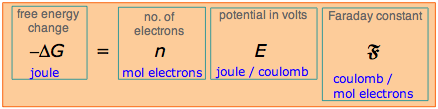A few remarks are in order about this very fundamental and important relation: The negative sign on the right indicates that a positive cell potential (according to the sign convention discussed previously) implies a negative free energy change, and thus that the cell reaction will spontaneously proceed to the right. Electrical work is done when an electric charge q moves through a potential difference ΔV. The right side of Eq. 2 refers to the movement of n moles of charge across the cell potential E°, and thus has the dimensions of work. The value of ΔG° expresses the maximum useful work that a system can do on the surroundings. "Useful" work is that which can be extracted from the cell by electrical means to operate a lamp or some other external device. This excludes any P-V work that is simply a consequence of volume change (which could conceivably be put to some use!) and which would be performed in any case, even if the reactants were combined directly. This quantity of work –ΔG° can only be extracted from the system under the limiting conditions of a thermodynamically reversible change, which for an electrochemical cell implies zero current. The more rapidly the cell operates, the less electrical work it can supply. If the Faraday constant F is expressed in coulombs per mole of electrons, the electrical work is in joules per mole. To relate these units to electrical units, recall that the coulomb is one amp-sec, and that power, which is the rate at which work is done, is measured in watts, which is the product of amps and volts:

1 J = 1 watt-sec = 1 (amp-sec) × volts(3-2)

Thus the volt has the dimensions of joules/coulomb– the energy produced per quantity of charge passing through the cell. Because voltage is the quotient of two extensive quantities, it is itself intensive. When we multiply the anodic and cathodic half-reactions by the stoichiometric factors required to ensure that each involves the same quantity of charge, the free energy change and the number of coulombs both increase by the same factor, leaving the potential (voltage) unchanged. This explains why we do not have to multiply the s of the anode and cathode reactions by stoichiometric factors when we are finding the potential of a complete cell.

If Eq. 2 is solved for , we have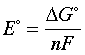(3-3)

This states explicitly that the cell potential is a measure of the free energy change per mole of electrons transferred, which is a brief re-statement of the principle explained immediately above.

To see this more clearly, consider the cell

Cu(s) | Cu2+ || Cl | AgCl(s) | Ag(s)(3-4)

for which we list the standard reduction potentials and ΔG°s of the half-reactions:

reaction -nFE°= ΔG°

cathode: 2 × [AgCl(s) + e → Ag(s) + Cl]

anode: Cu(s) → Cu2+(aq) + 2 e

net: 2 Ag(s) + 2 Cl(aq) + Cu2+(aq) → AgCl(s) + Cu(s)

+0.22 v

–(+.337) v

–.115 v

–42800 J

+65000 J

+22200 J

Notice that we multiply the cathodic reaction by two in order to balance the charge, but this does not affect the value of for the half reaction. It does, however, double the value of n, and hence -nFE° in the rightmost column.

Because the anodic reaction is written as an oxidation, we reverse the sign of its and obtain Ecell = Eright – Eleft = –.115 volt for the cell potential. The negative cell potential tells us that this reaction will not proceed spontaneously.

### When the electrons don't cancel out

Note, however, that if we are combining two half reactions to obtain a third half reaction, the values are not additive, since this third half-reaction is not accompanied by another half reaction that causes the charges to cancel. But free energies are always additive, so we can calculate their values, add them, and use ΔG° =  –nFE° to find the cell potential.

4  The fall of the electron

A table of standard half-cell potentials summarizes a large amount of chemistry, for it expresses the relative powers of various substances to donate and accept electrons by listing reduction half-reactions in order of increasing E° values, and thus of increasing spontaneity. The greater the value of E°, the greater the tendency of the substance on the left to acquire electrons, and thus the stronger this substance is as an oxidizing agent.

If you have studied elementary chemical thermodynamics, you will have learned about the importance of a quantity called the Gibbs free energy (usually referred to as simply the "free energy") in determining the direction of any chemical change. The rule is that all spontaneous change (that is, any reaction that proceeds to the "right") is associated with a fall in the free energy, and the greater the degree of that fall (Δ), the greater will be the tendency for the reaction to take place.

If you are not familiar with the concept of free energy, just think of it as something like potential energy, which similarly decreases when spontaneous mechanical events occur, such as the dropping of a weight.

Since oxidation-reduction processes involve the transfer of one or more electrons from a donor to an acceptor, it makes sense to focus on the electron and to consider that it falls from a higher-free energy environment (the reductant, or "source") to a lower-free energy one (the oxidant, or "sink".)

### Anatomy of an electron-free energy chart

Consider the simple electron-transfer reaction we have dealt with earlier, in which the more "active" zinc "displaces" copper(II) ion from solution:

Zn(s) + Cu2+ → Zn2+ + Cu(s)

This reaction involves the two couples Zn2+/Zn and Cu2+/Cu, whose standard reduction potentials are shown in green print along the center vertical line on the plot. Because these E° values are with respect to the standard hydrogen electrode, the H+/H2 couple is also shown.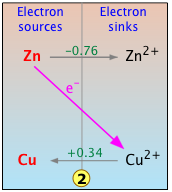Now, in this simpified chart,we focus on the reaction of interest. The diagonal line represents the "fall of the electron" from its free energy at the zinc level to that at the copper level. This process is made possible by the "electron vacency" present in the electron-sink Cu2+. The results of this transformation are indicated by the arrows that appear on the gray horizontal lines that connect each couple, which correspond to the reaction
Zn(s) + Cu2+ → Zn2+ + Cu(s) .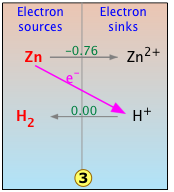Of course, Zn, which is one of the more "active" metals near the top of the electromotive series, can be reduced by any number of the other electron-sink cations that fall below it. For example, Zn will react with acid to liberate hydrogen gas as shown here.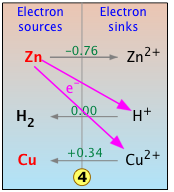What happens if we drop some metallic zinc into an acidic solution of copper(ii) nitrate?  The answer depends on the relative concentrations of the two electron acceptors H+ and Cu2+. In general, the lowest electron sink will receive most of the electrons, with a smaller proportion ending up on any higher electron sinks that may be available.  This proportion depends not only on the relative concentration of the accepting species, but also on their vertical separation; the greater the free energy difference (and thus the difference in the E values of the two couples,) the more strongly will the lowest sink be favored.

The details of how these calculations are made will be explored in the lesson on the Nernst equation, which follows the present one.

### Electron-free energy chart of standard reduction potentials

Visual representations of numeric data such as s can reveal trends or relationships that might otherwise not be apparent to us. In  this example, you see a selected series of redox couples in relation to entire range of commonly encountered E° values within the interval &plusmin;3 volts. Here are a few points with noting: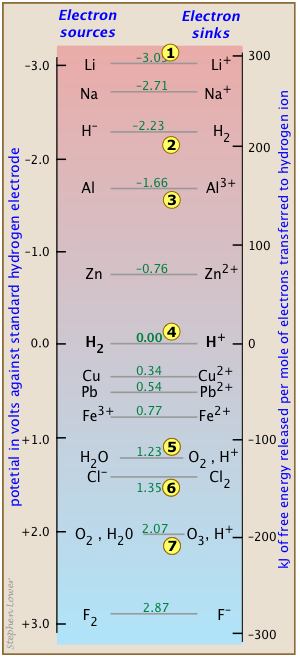Lithium's location at the top of the chart 1 reminds us of why it is such a favored component of batteries. Each mole of electrons it delivers to oxygen 4 yields around 400 kJ of energy. The light weight of this element is also a great advantage; imagine what it would be like to carry a calculator or iPhone sporting a lead battery! Oxygen 4 is the favored electron sink in fuel cells because it is freely available from the air. Batteries carry their own electron acceptors internally, commonly employing metal oxides rather than elemental oxygen. But O2 can also play the villian when it comes to corrosion; it can swallow up electrons from every metal above it. You might wonder why an active metal like aluminum 3 can survive in the atmosphere without corroding away.  The answer is that its surface does react quite rapidly with oxygen, but the corrosion product Al2O3 forms an unusually adherent layer that protects interior of the structure from further corrosion. The H2/H2couple is of course the basis of the standard hydrogen electrode, but it also marks the borderline between the "active" metals that can "displace hydrogen" from acids. Thus metallic zinc, or any metal above it, will lose electrons to the H+ ions 4 in equilibrium with water at pH zero (effective [H+] = 1M,) liberating hydrogen in the process. The couple 5 is by far the most important one on this chart because it dominates the oxygenated environment, including most the biosphere. As the ultimate electron acceptor of the natural world, O2 is the reason that the metals exist as oxide ores. 5 also marks a significant boundary: the point at which water becomes thermodyamically unstable. This means that any electron sink below it (such as Cl2 6) will tend to decompose water. Finally, 7 reminds us that ozone O3 is a very strong oxidizing agent; being capable of sucking electrons out of water itself. The resulting gradual destruction of O3 where it is used to disinfect water can be a problem by the time the water makes its way to locations distant from the treatment plant.

An important reminder: themodynamics only points the way to equilibrium, but says nothing about the rate of a process. Electron-transfers involving gaseous hydrogen and oxygen can be notoriously slow and dependent on the nature of the electrode. For example, a piece of really pure zinc metal can happily reside in hydrochloric acid indefinitely without any observable change, but throw in a bit of impure zinc, and it rapidly disappears in a fizzy hydrogen bubble bath.

And one other thing: don't be misled into regarding the metallic elements such as copper and lead as "inactive" simply because they fall below 4 and don't displace H2 from water.  The equilibrium constant for the reaction
Pb(s) + 2 H+ → Pb2+ + H2 may be very small, but it is neverthless enough to have endangered the lives of many people.

5  Latimer diagrams

Need some review on oxidation states/numbers?

Considerable insight into the chemistry of a single element can be had by comparing the standard electrode potentials (and thus the relative free energies) of the various oxidation states of the element. The most convenient means of doing this is the Latimer diagram. As an example, consider the Latimer diagram for iron: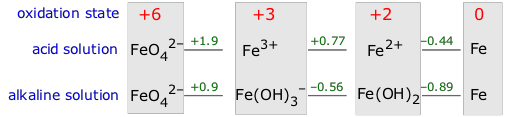Cl2 can oxidize water (green arrows, top) and also undergo disproportionation (purple arrows, bottom). In the latter process, one Cl2 molecule donates electrons to another.
6  Limitations on electrical work done by galvanic cells

The free energy change for a process represents the maximum amount of non-PV work that can be extracted from it. In the case of an electrochemical cell, this work is due to the flow of electrons through the potential difference between the two electrodes. Note, however, that as the rate of electron flow (i.e., the current) increases, the potential difference must decrease; if we short-circuit the cell by connecting the two electrodes with a conductor having negligible resistance, the potential difference is zero and no work will be done. The full amount of work can be realized only if the cell operates at an infinitesimal rate; that is, reversibly.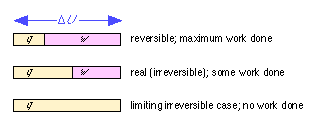You should recall that this is exactly analogous to the expansion of an ideal gas. The full amount of work w = PdV is extracted only under the special condition that the external pressure P opposing expansion is only infinitesimally smaller than the pressure of the gas itself. If the gas is allowed to expand into a vacuum (P = 0), no work will be done.

The total amount of energy a reaction can supply under standard conditions at constant pressure and temperature is given by ΔH°. If the reaction takes place by combining the reactants directly (no cell) or in a short-circuited cell, no work is done and the heat released is ΔH. If the reaction takes place in a cell that performs electrical work, then the heat released is diminished by the amount of electrical work done. In the limit of reversible operation, the heat released becomes

ΔH = Δ + T ΔS